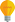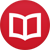# Second substitution method for integration without variables

by Jakub MarianTip: See my list of theMost Common Mistakes in English. It will teach you how to avoid mis­takes with com­mas, pre­pos­i­tions, ir­reg­u­lar verbs, and much more.

This article is based on my article about integration without variables which you may want to read first in order to understand what’s going on here. We will assume in the whole article that the integrals are being solved only on an appropriate interval, so that all inverse functions that might arise are well defined.

There are two methods traditionally used for integration by substitution. Say, you have an integral $∫f(x)dx$. The most usual method is to write $t = g(x)$ for some $g$, express “$f(x)dx = h(t)dt$”, compute $∫h(t)dt$, and substitute $g(x)$ back for $t$. Of course, this is not a rigorous process; its rigorous version can be expressed using a notation without variables:

$$∫f(g)g’ = \l(∫f\r)(g)$$

where the symbol $f(g) = f ∘ g$ denotes composition of functions. There is also another version of the substitution method. Instead of $t = g(x)$, we can set $x = g(t)$. For example, traditionally, we could solve the integral $∫√{1-x^2}dx$ using $x = \sin(t)$ as follows:

$$∫ √{1-x^2}\,dx = ∫ √{1-\sin^2(t)}\,d(\sin(t)) = ∫ \cos(t)\cos(t)\,dt = ÷{1}{2}(\cos(t)\sin(t)+t)+c$$

Here we used the formula for the integral of $\cos^2(t)$ and now have to substitute $x = \sin(t)$, i.e. $t = \arcsin(x)$, back:

$$÷{1}{2}(\cos(t)\sin(t)+t)+c = ÷{1}{2}(√{1-\sin^2(t)}\sin(t)+t)+c = ÷{1}{2}(x√{1-x^2}+\arcsin{x})+c$$

Yet again, this is not a rigorous way to perform the computation.

## The second substitution method without variables

The second substitution method has a really simple formulation in the notation without variables:

$$∫ f = \l(∫f(g)g’\r)(g⁻¹)$$

where $g$ is some invertible function. How to see it? It trivially follows from the previous substitution method:

$$\l(∫f(g)g’\r)(g⁻¹) = \l(∫f\r)∘g∘g⁻¹ = ∫f$$

How does it work in a particular example?

$$∫ √{1-\id^2} = \l(∫√{1-\sin^2}{\cos}\r)(\arcsin) = \l(∫{\cos \cos}\r)(\arcsin) = ÷{1}{2}({\cos \sin}+\id)(\arcsin) + C = ÷{1}{2}\l(√{1-\sin^2}{\sin}+\id\r)(\arcsin) + C = ÷{1}{2}\l(\id√{1-\id^2}+\arcsin\r)+C$$

This solution is completely rigorous, done using only simple algebraic manipulation and without any substitution at all.

By the way, I have written several educational ebooks. If you get a copy, you can learn new things and support this website at the same time—why don’t you check them out?